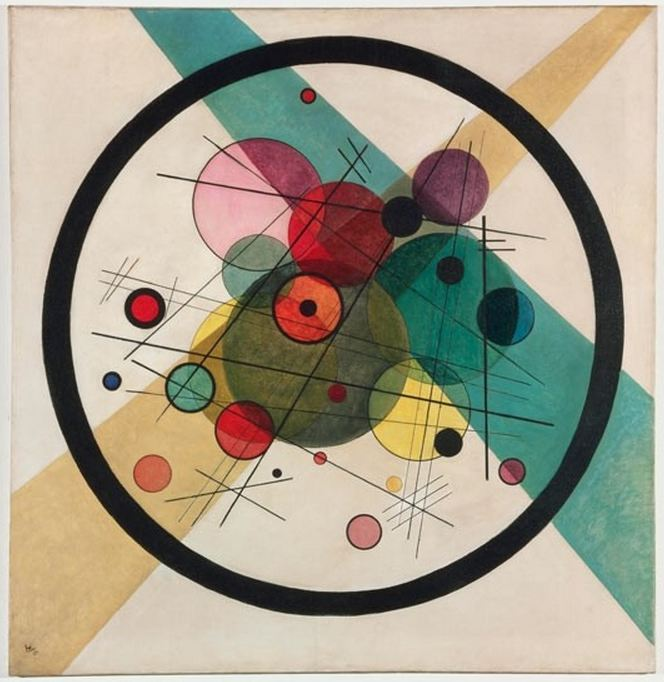W. Kandinsky: Circles in a Circle, 1923

# Circles

We are given a set $$\cal C$$ of $$m$$ circles in the plane $${\mathbb R}^2$$.
1. Design an algorithm that tests if all circles in the set $$\cal C$$ lie inside one of the circles.
2. Design an algorithm that reports all pairs of intersecting circles.
3. Design an algorithm that outputs a directed graph $$\cal D$$ on $$\cal C$$, in which there is an edge from $$C_1$$ to $$C_2$$ if circle $$C_1$$ lies inside circle $$C_2$$ and there is no circle $$C_3 \in \cal C$$ in between (note that $$\cal D$$ is a forest).
Prove the correctness of your algorithms and find their running times.